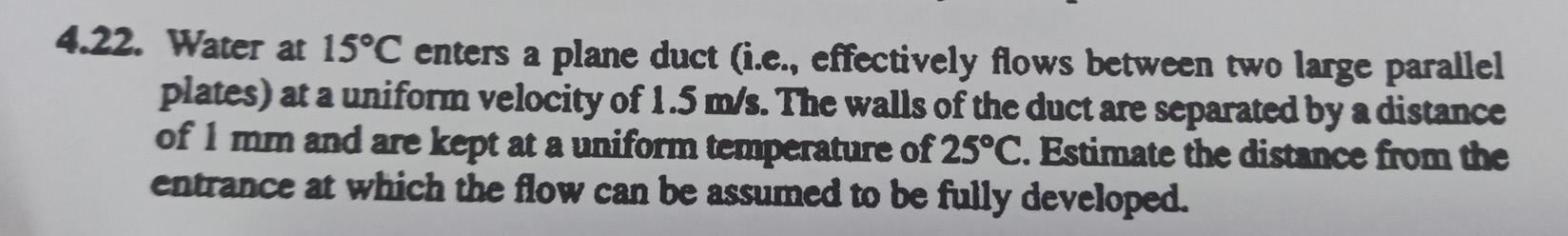# Question Solved1 Answer4.22. Water at 15°C enters a plane duct (i.c., effectively flows between two large parallel plates) at a uniform velocity of 1.5 m/s. The walls of the duct are separated by a distance of 1 mm and are kept at a uniform temperature of 25°C. Estimate the distance from the entrance at which the flow can be assumed to be fully developed.S72JB9 The Asker · Mechanical EngineeringTranscribed Image Text: 4.22. Water at 15°C enters a plane duct (i.c., effectively flows between two large parallel plates) at a uniform velocity of 1.5 m/s. The walls of the duct are separated by a distance of 1 mm and are kept at a uniform temperature of 25°C. Estimate the distance from the entrance at which the flow can be assumed to be fully developed.
More
Transcribed Image Text: 4.22. Water at 15°C enters a plane duct (i.c., effectively flows between two large parallel plates) at a uniform velocity of 1.5 m/s. The walls of the duct are separated by a distance of 1 mm and are kept at a uniform temperature of 25°C. Estimate the distance from the entrance at which the flow can be assumed to be fully developed.